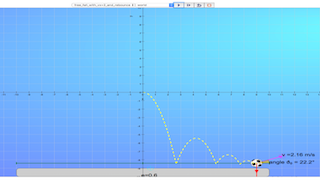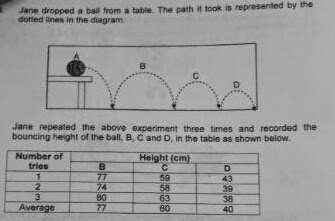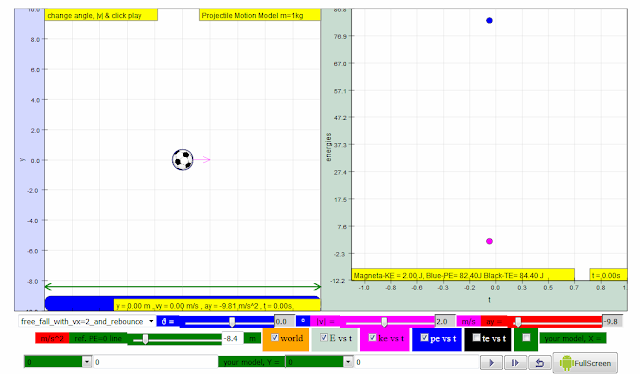## Topics

Energy forms
Energy conversion

## Takeaways:

Energy makes changes and movement possible in everyday life
Energy is required to enable things to work or move.
There are different forms of energy and they can be converted from one form to another.

## Description

This simulation allow students to explore the concepts of kinetic energy and potential energy. When the re-bounce case is selected, the sum of kinetic energy and potential energy drop immediately after each contact with the ground. The energy loss is typically associated with sound and heat energy loss to the surrounding by the ball.

## Sample Learning Goals

**Recognise and give examples of the various forms of energy. - kinetic energy - potential energy
**Investigate energy conversion from one form to another and communicate findings.

## Version:

Projectile Motion Model

### Translations

Code Language Translator Run### Software Requirements

SoftwareRequirements

 Android iOS Windows MacOS with best with Chrome Chrome Chrome Chrome support full-screen? Yes. Chrome/Opera No. Firefox/ Samsung Internet Not yet Yes Yes cannot work on some mobile browser that don't understand JavaScript such as..... cannot work on Internet Explorer 9 and below

### CreditsThis email address is being protected from spambots. You need JavaScript enabled to view it.

### end faq

http://iwant2study.org/lookangejss/02_newtonianmechanics_7energyworkpower/ejss_model_projectileprimary/projectileprimary_Simulation.xhtml

### Sample Learning Goals### For Teachers

Activity 1

INVESTIGATE

Go to the following simulation: http://tinyurl.com/ast2016-3The left panel shows a football being given an initial velocity. This could be zero (the ball is being dropped), upwards (tossed up) or horizontal (thrown horizontally).

The right panel allows you to plot the energy of the object over time. The default is a plot for kinetic energy (ke), but you can also request potential energy (pe), and total energy (te). Remember that you can play the simulation by clicking the appropriate button (►). Click the pause button ( ▌▌) to pause the simulation.

1. First select the “free_fall” setting, using the yellow drop-down box in the bottom panel. Watch the ke and pe graphs as the object moves. What do you notice about the graphs?
• suggested answer: kinetic energy increases from t = 0 , KE = 0 J at an increasing rate. Potential energy decreases from t = 0 s, PE = maximum J at an decreasing rate as shown in the sketch.2. Now try the “toss_up” setting. How are the ke and pe graphs different?
• suggested answer: kinetic energy decreasing from t = 0 , KE = 50 J at an decreasing rate until t =1 s. From t >1 s,  Kinetic energy increases at an increasing rate. Potential energy increases from t = 0 s to 1 s. From t>1 s, PE decreases at decreasing rate as shown in the sketch.3. Select total energy te checkbox, suggest an big idea relating ke and pe to te.
• suggested answer: ke + pe = te4. You can alter the reference line for this simulation. Reset the simulation for “free_fall” or for “toss_up” but, before you press play, change the “ref. PE=0 line” to a different value.
How are the ke and pe graphs different when you choose a different PE reference line?
• suggested answer: reference PE = 0 is a imaginary line for which potential energy is calculated referencing from. ke is not affected by “ref. PE=0 line”MODEL

You may have noticed that the kinetic energy ke depends on the speed |v|. Now set the simulation to “user_defined”. You can choose the speed you want by typing the value in the magenta box in the bottom. You can read the corresponding kinetic energy ke in the yellow box below the graph in the right-hand panel. Try to fill up the table below.

 Speed |v| in m/s Speed x Speed (v2) Kinetic energy ke in J 0.00 1.00 2.00 3.00 9.00 4.00 5.00 12.5

Now that you have investigated some of the different motions of the football, let’s try to model this by creating some rules about pe and ke. Note that the mass of the ball in the simulation is 1.00 kg, as indicated in the yellow box above the image on the left.

by observing the data is the table, suggest a viable equation-model for ke in terms of v.

1. Write down some rules for the relationships between pe and ke. Here’s an example:

ke + pe = te

the value of pe is measured from the PE reference line

“The graph for ke does not depend on the PE reference line.”

APPLY

Now try to test your model to see if your rules hold true for a different pre-set motion: “free_fall_and_rebounce”.

1. Which of your rules are still the same after trying the “free_fall_and_rebounce” setting?
• ke +pe = te
• the value of pe is measured from the PE reference line
2. Which of the rules were not retained?
• ??, ke = 0.5*v^2 is incomplete, the complete equation is ke = 0.5*m*v^2 where m is the mass of the object.

### Energy transfers and transformations Key inquiry question:

How can we account for the ‘appearance’ and ‘disappearance’ of energy when objects interact with each other?

#### 1. & 2.Work and power

• Work done is defined as the force x distance moved in the direction of the force.

• When we do work, energy is ‘used up’. The amount of work is equal to the energy transferred or used.

• Power is the rate of doing work and is given by: power = work done / time taken (P = W/t).

#### 3. Potential energy and kinetic energy

• Kinetic energy is the energy a body possesses due to its motion.

• Kinetic energy Ek = ½ mv2 .

• Potential energy is the energy stored in a body due to its position, state or shape (e.g. elastic, gravitational, chemical). Gravitational potential energy is the energy which a body possesses due to its position relative to the ground. Gravitational potential energy Ep = mgh.

• The total mechanical energy (Ek and E p) is conserved if no frictional forces are present in a moving system.

#### 4. Energy conversion and conservation

• Energy is defined as the capacity to do work. Energy is transferred when work is done.

• There are different forms of energy e.g. kinetic energy, elastic potential energy, gravitational potential energy, chemical potential energy and thermal energy.

• Energy can be transformed from one form to another but cannot be destroyed or created (principle of conservation of energy).

### Students’ prior knowledge of Energy

#### Primary level:

Students learn that energy:

• is required to make things work or move and energy from most of our energy resources is derived in some ways from the Sun, our primary source of light and heat energy.

• exist in different forms e.g. kinetic energy (movement energy), gravitational potential energy (objects above the ground), elastic potential energy (spring, elastic band), light energy, electrical energy, sound energy, heat energy and chemical energy (as a form of stored energy: food, batteries, fuels).

Students do simple experiments to investigate energy conversion from one form to another e.g. in an electric circuit; learn about sources of energy such as wind, water and fuels, and the need to reduce energy usage in our everyday lives.

#### Lower secondary level:

Students learn that:

• work is the use of a force to move an object.

• work done by a force is defined as force x distance moved in the direction of the force and, based on this definition of work, there are situations involving forces where work is done and where work is not done (calculation only for force parallel to direction of motion) (unit: joule).

• energy is the ability (or capacity) to do work or to produce change (work done = energy used) and there are different forms of energy e.g. kinetic, potential, light and sound.

• energy is conserved and can only change from one form to another (the total amount of energy before and after the change is exactly the same).

• sources of energy include fossil fuels (coal, oil, gas), kinetic energy from water and wind, nuclear, solar, and biomass.

### Students’ common misconceptions and learning difficulties in Energy

Forces between masses, charges and magnets:

Students often think of energy either as a physical substance that flows out of one thing to another or as a kind of force. Work done on a body: Students have difficulty understanding that the work done on a body represents the energy transferred during the interaction between the body and another system, and does not represent energy stored in a body.

Energy is conserved:

Students often think that energy is used up or lost (disappears) during interactions

Research

[text]

[text]

### Project related:

Understanding Teacher Learning Community as Support for Implementation of Open Source Physics for Conceptual Instruction
Project Number: OER 10/15 GWF
Project Duration: 01 July 2015 - 30 April 2017

### end faq